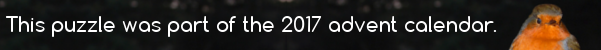mscroggs.co.uk
mscroggs.co.uksubscribe

# Puzzles

## 11 DecemberThis puzzle is inspired by a puzzle Woody showed me at MathsJam.
Today's number is the number $$n$$ such that $$\frac{216!\times215!\times214!\times...\times1!}{n!}$$ is a square number.

## 4 DecemberToday's number is the number of 0s that 611! (611×610×...×2×1) ends in.

## 10 DecemberHow many zeros does 1000! (ie 1000 × 999 × 998 × ... × 1) end with?

## Factorial pattern

$$1\times1!=2!-1$$ $$1\times1!+2\times2!=3!-1$$ $$1\times1!+2\times2!+3\times3!=4!-1$$
Does this pattern continue?

## Square factorials

Source: Woody at Maths Jam
Multiply together the first 100 factorials:
$$1!\times2!\times3!\times...\times100!$$
Find a number, $$n$$, such that dividing this product by $$n!$$ produces a square number.

## 17 DecemberIn March, I posted the puzzle One Hundred Factorial, which asked how many zeros 100! ends with.
What is the smallest number, n, such that n! ends with 50 zeros?

## One hundred factorial

How many zeros does $$100!$$ end with?

## Archive

Show me a random puzzle
▼ show ▼